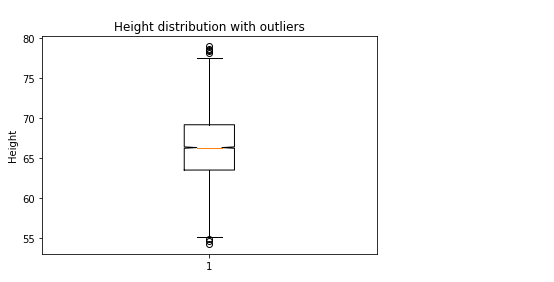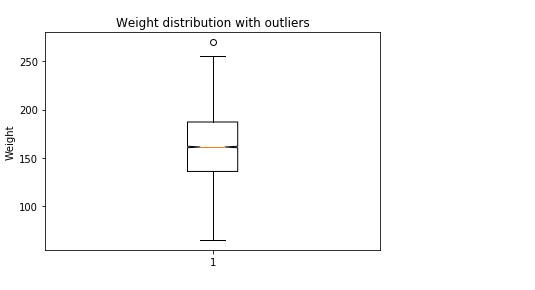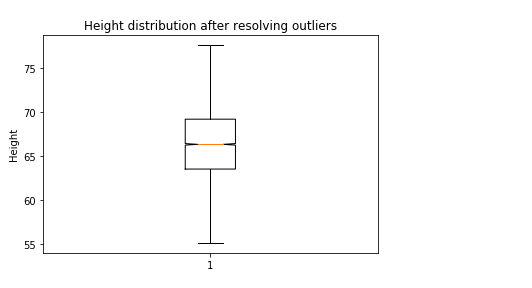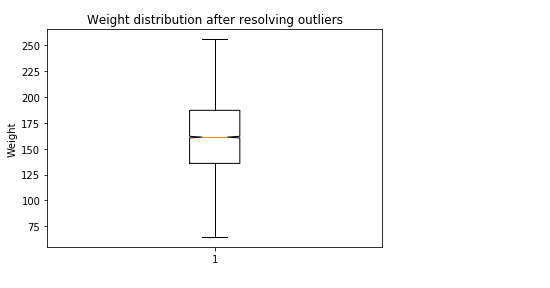• #5, First Floor, 4th Street Dr. Subbarayan Nagar Kodambakkam, Chennai-600 024 Landmark : Samiyar Madam
• pro@slogix.in
• +91- 81240 01111

How to create simple linear regression model for the data set height and weight of male and female in python?

Description

To create a simple linear regression model for the given data set and analyse the summary and goodness of the model

Process

Find and resolve the Missing values

Find and resolve the Outliers

Split the data set for training and testing with ratio 80:20 so that training and testing data has 80% and 20% of the original data set respectively

Build the model

Fit the model using the training data

Test the model using the test data

Take the summary and analyse it

Building a simple linear regression model :

Simple linear regression model can be created in python in two different methods.They are

Using sklearn library

Using statsmodel api

Using sklearn library : Required libraries :

from sklearn.model_selection import train_test_split

from sklearn.linear_model import LinearRegression

from sklearn import metrics

Functions used :

To split the data - x_train, x_test, y_train, y_test = train_test_split(x, y, test_size=0.20, random_state=42)

To build the model - reg=LinearRegression()

To train the model - reg.fit(x_train_data,y_train_data)

To test the model - reg.predict(x_test_data)

To find the R -Squared value - reg.score(x_test,y_test)

To find the R-Squared value using metrics library - metrics.r2_score(y_test,y_pred)

Using statsmodel api : Required libraries :

import statsmodels.api as sm

Functions used :

To Train the model - model = sm.OLS(y_train,x_train)

To fit the model - results = model.fit()

To test the model - results.predict(x_test)

To take the summary -results.summary()

Sapmle Code

#import necessary libraries
import pandas as pd
import numpy as np
import matplotlib.pyplot as plt
from sklearn.model_selection import train_test_split
from sklearn.linear_model import LinearRegression
from sklearn import metrics
import statsmodels.api as sm
import warnings
warnings.filterwarnings(“ignore”)
#Function to find and resolve the missing values
def res_mv(df):
for i in df.describe().columns:
if df[i].isnull().sum()!=0:
df[i].fillna(df[i].median(),inplace=True)
for i in df.describe(include=pd.core.series.Series).columns:
if df[i].isnull().sum()!=0:
df[i].fillna(method=”bfill”,inplace=True)
#Function to detect and resolve the outliers
def outlier_detect(df):
for i in df.describe().columns:
Q1=df.describe().at[‘25%’,i]
Q3=df.describe().at[‘75%’,i]
IQR=Q3 – Q1
LTV=Q1 – 1.5 * IQR
UTV=Q3 + 1.5 * IQR
x=np.array(df[i])
p=[]
for j in x:
if j < LTV or j>UTV:
p.append(df[i].median())
else:
p.append(j)
df[i]=p
print(“Outliers resolved”)
return df
#To Resolve the Missing values
res_mv(data)
#To identify the outliers using boxplot
plt.boxplot(data[‘Height’],notch=True)
plt.title(‘Height distribution with outliers’)
plt.ylabel(‘Height’)
plt.show()

plt.boxplot(data[‘Weight’],notch=True)
plt.title(‘Weight distribution with outliers’)
plt.ylabel(‘Weight’)
plt.show()
#To Resolve the outliers
data=outlier_detect(data)
#boxplot after resolving the outliers
plt.boxplot(data[‘Height’],notch=True)
plt.title(‘Height distribution after resolving outliers’)
plt.ylabel(‘Height’)
plt.show()

plt.boxplot(data[‘Weight’],notch=True)
plt.title(‘Weight distribution after resolving outliers’)
plt.ylabel(‘Weight’)
plt.show()
#convert the dataset so that it has 1 dimension array with 0 features
x=data[[‘Height’]].values.reshape(-1,1)
y=data[‘Weight’].values.reshape(-1,1)
#data dplitting for training and testing the model
x_train, x_test, y_train, y_test = train_test_split(x, y, test_size=0.20, random_state=42)
#To Build the model using SkLearn
reg = LinearRegression()
reg.fit(x_train,y_train)
print(“Regression intercept is “,reg.intercept_)
print(“Regression coefficient is “,reg.coef_)
print(“The determination of coefficient R^2 is “,reg.score(x_test,y_test))
y_pred=reg.predict(x_test)
print(“The R^2 value for actual and predicted value is “,metrics.r2_score(y_test,y_pred))
print(reg.score(x_train,y_train))
#To Build the model using statsmodel pi
#Xtrain should be given to add constant() to see the coefficients
#using the statsmodel
#build regression model
model = sm.OLS(y_train,x_train)
results = model.fit()
#Take the summary of the model
print(“Summary of Linear regression model created using stats model api”)
print(results.summary())
#predict the y value using x test